# Breather Wave and Kinky Periodic Wave Solutions of One-Dimensional Oskolkov Equation

Breather Wave and Kinky Periodic Wave Solutions of One-Dimensional Oskolkov Equation

Mamunur Roshid Habibul Bashar*

Department of Mathematics, Pabna University of Science and Technology, Pabna 6600, Bangladesh

Corresponding Author Email:
hbashar27.1273@gmail.com
Page:
460-466
|
DOI:
https://doi.org/10.18280/mmep.060319
14 April 2019
|
Accepted:
2 July 2019
|
Published:
15 September 2019
| Citation

OPEN ACCESS

Abstract:

In this work, we confer the propagation of nonlinear Kinky periodic wave and breather wave for the dominant nonlinear pseudo-parabolic physical models: the one-dimensional Oskolkov equation is explored. By executing simple equation method, compilation of disguise adaptation of exact nonlinear wave solutions with some noteworthy parameters for the Oskolkov equations is accessible. The elements of acquired nonlinear wave arrangements are broke down and delineated in figures by choosing suitable parameters. The simple equation scheme is solid treatment for seeking fundamental nonlinear waves that improve assortment of dynamic models emerges in designing fields. The presentation of this technique is dependable, direct, and easy to execute contrasted with other existing strategies.

Keywords:

kinky periodic wave, breather wave, the Oskolkov equation, simple equation method

1. Introduction

In the last few decennary, the study of nonlinear evolution equations established much concentration in diverse fields of nonlinear science, such as fluid mechanics, nuclear physics, solid-state physics, plasma physics, chemical physics, optical fibre and geochemistry. Many scholars planned through NEEs to construct traveling wave solution by implement several methods. The procedures that are well established in recent literature such as extended Kudryashov method , Modefied simple equation method ,  New extended (G’/G) expansion method [3-4], Darboux transformation , trial solution method , Exp-Function Method , Multiple Simplest Equation Method . In this paper we implement simple equation method to execute innovative traveling wave solution. Nofal applied Simple equation method for nonlinear partial differential equations . Diverse authors are solved some numerous models by simple equation method [10-13].

Pseudo parabolic model is one kind of partial differential equations in which the time derivative emerged in highest order derivative and they have been exploiting for different areas of mathematics and physics such as instance, for fluid flow in fissured rock, consolidation of clay, shear in second-order fluids, thermodynamics and propagation of long waves of small amplitude. Nowadays, much attention has been paid to investigate NEEs such as Pseudo parabolic model [15-20]. It is important to note that a completely integrable Pseudo parabolic model provides innovative and explicit different type exact traveling wave solution.

In the present work, we reflect on the one dimensional Oskolkov equation. Implementing the simple equation method, we attain the kinky periodic wave and breather wave solutions. We utilize numerical reproduction to ponder the one dimensional Oskolkov equation. We consider (1+1) Dimensional Oskolkov Equation in the following form

$U_{t}-\beta U_{x x t}-\alpha U_{x x}+U U_{x}=0$      (1)

This equation is pseudoparabolic equation and one-dimensional analogue of the oskolkov system

$\left(1-\lambda \nabla^{2}\right) U_{t}=\alpha \nabla^{2} U-(U \bullet \nabla) U-\nabla^{2} p+f$, where $\nabla \bullet U=0$.         (2)

This system illustrates the dynamics of an incompressible viscoelastic Kelvin-Voigt fluid. It was indicated in [20-21] that the parameter λ can be negative and the negativeness of the parameter λ does not deny the physical meaning of equation (2). We implemented the simple equation method to solve equation (1) and obtained new solutions which could not be attained in the past. Mamunur found exact and explicit solution from Oskolkov equation with the help of MSE method , Faruk applied the tanh-coth method for some nonlinear pseudo parabolic equations to obtained exact solution , Turgut Propagation of nonlinear shock waves for the generalized oskolkov equation and its dynamic motions in the presence of an external periodic perturbation by implement inified method  and others author solve this model by different dominant method [14-20].

The objective of this article is to pursue new study for anticipate to the simple equation method to explore wave solutions of Oskolkov equations. The principal convenience of this method over the existing other methods is that it provides more new exact traveling wave solutions.

2. Methodology

The methodology of the simple equation method used up to now is appropriate for solving model nonlinear PDEs. The extension of the methodology is presented in the following subsection

$\mathfrak{R}\left(u, u_{t}, u_{x}, u_{t t}, u_{x x}, u_{x t} \ldots \ldots \ldots\right)=0$ ,    (3)

where $u(\xi)=u(x, t)$  is an unknown function, $\mathfrak{R}$ is a polynomial of $u(x, t)$  and its partial derivatives in which the highest order derivatives and nonlinear terms are involved. The accompanying advances are engaged with finding the arrangement of nonlinear Equation (3) utilizing this strategy.

Step 1: The given PDE (3) can be changed into ODE utilizing the change $\xi=x \pm \omega t$  , where $\omega$ is the speed of traveling wave such that $\omega \in R-\{0\}$ .

The traveling wave transformation permits us to reduce Eq. (3) to the following ODE:

$\mathfrak{R}\left(u, u^{\prime}, u^{\prime \prime}, \ldots \ldots \ldots \ldots\right)=0$ ,       (4)

where $\mathscr{R}$ is a polynomial in $u(\xi)$ and its derivatives, where  $u^{\prime}(\xi)=\frac{d u}{d \xi}, u^{\prime \prime}(\xi)=\frac{d^{2} u}{d \xi^{2}}$  and so on.

Step 2. We seek the solution of Eq. (3) in the following form:

$U(\xi)=\sum_{i=0}^{n} a_{i} F(\xi)^{i}$       (5)

where $a_{i}(i=0,1,2, \ldots . N)$  are arbitrary constants to be determined later and $F(\xi)$  satisfies some trial simple equation. The simple equation has two properties, the first one it is of lesser order than Eq. (5) and the second its general solution is known.

In this paper, the simple equations we shall utilize are the Riccati and Bernoulli differential equations which are surely understood nonlinear ordinary differential equations, and their solutions can be communicated by elementary functions.

Step-3. We recognize the positive integer $N$  in Eq. (5) by considering into account the homogeneous balance between the highest order derivatives and the nonlinear terms in Eq. (5). Moreover the degree of $U$ as $D(U(\xi))$ which gives the order of others expression as follows

$D\left(\frac{d^{q} U}{d \xi^{q}}\right)=N+q, D\left(U^{p}\left(\frac{d^{q} U}{d \xi^{q}}\right)^{s}\right)=s N+s(N+q)$

Therefore we can obtain the value of $N$ in equation (5) with the guide of above formula.

Step-4. We discuss the general solutions of the simplest equation method. For the Bernoulli equation

$F^{\prime}(\xi)=R F(\xi)+h F^{2}(\xi)$       (6)

where $R$ and $h$  are arbitrary constants.

The general solution of Eq. (6) takes the following form:

$F(\xi)=\frac{\operatorname{Re} x p\left[R\left(\xi+\zeta_{0}\right)\right]}{1-h \exp \left[R\left(\xi+\xi_{0}\right)\right]}, h<0, R>0$

where $\xi_{0}$ is the integration constant and

$F(\xi)=-\frac{\operatorname{Re} x p\left[R\left(\xi+\zeta_{0}\right)\right]}{1+h \exp \left[R\left(\xi+\xi_{0}\right)\right]}, h<0, R>0$

Remark:

When $R=\delta$ and $h=-1$, Eq. (6) has another form the Bernoulli equation

$F^{\prime}(\xi)=\delta F(\xi)-F^{2}(\xi)$

The general solution of Eq. (6) takes the following

$F(\xi)=\frac{\delta}{2}\left[1+\tanh \left(\frac{\delta}{2}\left(\xi+\xi_{0}\right)\right)\right],$ where $\delta>0$ and

$F(\xi)=\frac{\delta}{2}\left[1-\tanh \left(\frac{\delta}{2}\left(\xi+\xi_{0}\right)\right)\right],$ where $\delta<0$

For the Riccati equation

$F^{\prime}(\xi)=c F^{2}(\xi)+d$         (7)

The general solutions of Eq. (7) take the following form

When $c d<0$ and $\xi_{0}$  is a positive number

$F(\xi)=-\frac{\sqrt{-c d}}{c} \tanh \left(\sqrt{-c d} \xi-\frac{v \ln \left(\xi_{0}\right)}{2}\right)$

When $c d>0$ and $\xi_{0}$  is a real number

$F(\xi)=\frac{\sqrt{c d}}{c} \tan \left(\sqrt{c d}\left(\xi+\xi_{0}\right)\right)$

3. Application

In this section we execute the simple equation method on (1+1) dimension Oskolkov equation to evaluate new innovative traveling wave solution. The (1+1) dimensional Oskolkov equation describe incompressible viscoelastic Kelvin-Voigt fluid in the following from

$U_{t}-\beta U_{x x t}-\alpha U_{x x}+U U_{x}=0$        (8)

where β,α are arbitrary constant and U(x,t) is an unknown function. Using the traveling wave variable U(x,t)=U(ξ) and ξ=kx-ωt where k is a constant and $\omega$  is wave speed. Now we transfigure the Eq. (8) into the accompanying Ordinary differential equation.

$2 k^{2} \omega \beta U^{\prime \prime}-2 \alpha k^{2} U^{\prime}-2 \omega U+k U^{2}=0$ ,      (9)

where the prime denote the derivative with respect to ξ.

According to step-3, the balance number between the linear term $U^{\prime \prime}$ and the nonlinear term $U^{2}$ is N=2. So the solution of the Eq. (9) is the following form

$U(\xi)=a_{0}+a_{1} F(\xi)+a_{2}(F(\xi))^{2}$.        (10)

Differential Eq. (10) with respect to  with the guide of Eq. (6) then we get

$U^{\prime}=a_{1} R F(\xi)+\left(a_{1} h+2 a_{2} R\right) F^{2}(\xi)+2 h a_{2} F^{3}(\xi)$

$U^{\prime \prime}=a_{1} R^{2} F(\xi)+\left(4 R^{2} a_{2}+3 R h a_{1}\right) F^{2}(\xi)+\left(2 a_{1} h^{2}+10 h a_{2} R\right) F^{3}(\xi)+6 a_{2} h^{2} F^{4}(\xi)$

Substituting the value of $U, U^{\prime}, U^{\prime \prime}$ in the Eq. (9) and equating the coefficient of $F^{i}(\xi)$ are zero where  i=0,1,2......, then we get polynomials in the following,

$12 k^{2} \omega \beta a_{2} h^{2}+k a_{2}^{2}=0$

$-4 \alpha k^{2} a_{2} h+2 k a_{1} a_{2}+4 k^{2} \omega \beta a_{1} h^{2}+20 k^{2} \omega \beta a_{2} R h=0$

$-4 \alpha k^{2} a_{2} R-2 \omega a_{2}+8 k^{2} \omega \beta a_{2} R^{2}+2 k a_{0} a_{2}-2 \alpha k^{2} a_{1} h+k a_{1}^{2}+6 k^{2} \omega \beta a_{1} R h=0$

$2 k a_{0} a_{1}-2 \omega a_{1}+2 k^{2} \omega \beta a_{1} R^{2}-2 \alpha k^{2} a_{1} R=0$

$k a_{0}^{2}-2 \omega a_{0}=0$

Now we solve the polynomial with the guide of maple 18.  We gauge the resulting solution

$k=\frac{1}{6} \sqrt{\frac{-6}{\beta}}, \omega=\frac{\alpha}{5 \beta R}, a_{0}=a_{1}=0, a_{2}=-\frac{8}{5} \sqrt{\frac{-6}{\beta}} \alpha h^{2}$

$k=\frac{1}{R} \sqrt{\frac{1}{6 \beta}}, \omega=\frac{\alpha}{5 \beta R}, a_{0}=\frac{2 \alpha}{5 \sqrt{\frac{\beta}{6}}}, a_{1}=0, a_{2}=-\frac{8}{5} \sqrt{\frac{-6}{\beta}} \alpha h^{2}+$

$k=\frac{1}{R} \sqrt{\frac{-1}{6 \beta}}, \omega=-\frac{\alpha}{5 \beta R}, a_{0}=-\frac{2 \alpha}{5 \sqrt{\frac{\beta}{6}}}, a_{1}=\frac{24 \alpha h \sqrt{\frac{-1}{6 \beta}}}{5 R}, a_{2}=\frac{8}{5 R^{2}} \sqrt{\frac{-6}{\beta}} \alpha h^{2}+$

$k=\frac{1}{R} \sqrt{\frac{1}{6 \beta}}, \omega=-\frac{\alpha}{5 \beta R}, a_{0}=0, a_{1}=\frac{24 \alpha h \sqrt{\frac{-1}{6 \beta}}}{5 R}, a_{2}=\frac{12}{5 R^{2}} \sqrt{\frac{1}{6 \beta}} \alpha h^{2}$

Case-01: when

$k=\frac{1}{6} \sqrt{\frac{-6}{\beta}}, \omega=\frac{\alpha}{5 \beta R}, a_{0}=a_{1}=0, a_{2}=-\frac{8}{5} \sqrt{\frac{-6}{\beta}} \alpha h^{2}$

$U_{1,1}(\xi)=-\frac{8}{5} \sqrt{\frac{-6}{\beta}} \alpha h^{2}\left(\frac{r \exp \left[r\left(\xi+\xi_{0}\right)\right]}{1-d \exp \left[r\left(\xi+\xi_{0}\right)\right]}\right)^{2}, \quad h<0$ and $R>0$

$U_{1,2}(\xi)=\frac{8}{5} \sqrt{\frac{-6}{\beta}} \alpha h^{2}\left(\frac{r \exp \left[r\left(\xi+\zeta_{0}\right)\right]}{1+d \exp \left[r\left(\xi+\xi_{0}\right)\right]}\right)^{2}$

$h>0$ and $R<0$

If we set $R=\delta$ and $h=-1$, then the solution tern to the following form

\begin{aligned} U_{1,3}(\xi) &=-\frac{8}{5} \sqrt{\frac{-6}{\beta}} \alpha h^{2}\left(\frac{\delta}{2}\left[1+\tanh \left(\frac{\delta}{2}\left(\xi+\xi_{0}\right)\right)\right]\right)^{2}, \delta>0 \\ U_{1,4}(\xi) &=-\frac{8}{5_{0}} \sqrt{\frac{-6}{\beta}} \alpha h^{2}\left(\frac{\delta}{2}\left[1-\tanh \left(\frac{\delta}{2}\left(\xi+\xi_{0}\right)\right)\right]\right)^{2}, \delta<0 \end{aligned}

where

$\xi=\frac{1}{6} \sqrt{\frac{-6}{\beta}} x-\frac{\alpha}{5 \beta R} t$。

Case-02: when

$k=\frac{1}{R} \sqrt{\frac{1}{6 \beta}}, \omega=\frac{\alpha}{5 \beta R}, a_{0}=\frac{2 \alpha}{5 \sqrt{\frac{\beta}{6}}}, a_{1}=0, a_{2}=-\frac{8}{5} \sqrt{\frac{-6}{\beta}} \alpha h^{2}$

$U_{2,1}(\xi)=\frac{2 \alpha}{5 \sqrt{\frac{\beta}{6}}}-\frac{8}{5} \sqrt{\frac{-6}{\beta}} \alpha h^{2}\left(\frac{r \exp \left[r\left(\xi+\xi_{0}\right)\right]}{1-d \exp \left[r\left(\xi+\xi_{0}\right)\right]}\right)^{2} h<0$ and $R>0$

$U_{2,2}(\xi)=\frac{2 \alpha}{5 \sqrt{\frac{\beta}{6}}}+\frac{8}{5} \sqrt{\frac{-6}{\beta}} \alpha h^{2}\left(\frac{r \exp \left[r\left(\xi+\xi_{0}\right)\right]}{1+d \exp \left[r\left(\xi+\xi_{0}\right)\right]}\right)^{2} h>0$ and $R<0$

If we set R=δ and h=-1, then the solution tern to the following form

\begin{aligned} U_{2,3}(\xi)=& \frac{2 \alpha}{5 \sqrt{\frac{\beta}{6}}}-\frac{8}{5} \sqrt{\frac{-6}{\beta}} \alpha h^{2}\left(\frac{\delta}{2}\left[1+\tanh \left(\frac{\delta}{2}\left(\xi+\xi_{0}\right)\right)\right]\right)^{2}, \delta>0 \\ U_{2,4}(\xi)=& \frac{2 \alpha}{5 \sqrt{\frac{\beta}{6}}}-\frac{8}{5} \sqrt{\frac{-6}{\beta}} \alpha h^{2}\left(\frac{\delta}{2}\left[1-\tanh \left(\frac{\delta}{2}\left(\xi+\xi_{0}\right)\right)\right]\right)^{2}, \delta<0 \end{aligned}

where

$\xi=\frac{1}{R} \sqrt{\frac{1}{6 \beta}} x-\frac{\alpha}{5 \beta R} t$。

Case-03: when

$k=\frac{1}{R} \sqrt{\frac{-1}{6 \beta}}, \omega=-\frac{\alpha}{5 \beta R}, a_{0}=-\frac{2 \alpha}{5 \sqrt{\frac{\beta}{6}}}, a_{1}=\frac{24 \alpha h \sqrt{\frac{-1}{6 \beta}}}{5 R}, a_{2}=\frac{8}{5 R^{2}} \sqrt{\frac{-6}{\beta}} \alpha h^{2}$

$U_{3,1}(\xi)=-\frac{2 \alpha}{5 \sqrt{\frac{\beta}{6}}}+\frac{24 \alpha h \sqrt{\frac{-1}{6 \beta}}}{5 R}\left(\frac{r \exp \left[r\left(\xi+\xi_{0}\right)\right]}{1-d \exp \left[r\left(\xi+\xi_{0}\right)\right]}\right)+\frac{8}{5 R^{2}} \sqrt{\frac{-6}{\beta}} \alpha h^{2}\left(\frac{r \exp \left[r\left(\xi+\xi_{0}\right)\right]}{1-d \exp \left[r\left(\xi+\xi_{0}\right)\right]}\right)^{2}, h<0$ and $R>0$

$U_{3,2}(\xi)=-\frac{2 \alpha}{5 \sqrt{\frac{\beta}{6}}}-\frac{24 \alpha h \sqrt{\frac{-1}{6 \beta}}}{5 R}\left(\frac{r \exp \left[r\left(\xi+\zeta_{0}\right)\right]}{1+d \exp \left[r\left(\xi+\xi_{0}\right)\right]}\right)-\frac{8}{5 R^{2}} \sqrt{\frac{-6}{\beta}} \alpha h^{2}\left(\frac{r \exp \left[r\left(\xi+\zeta_{0}\right)\right]}{_{1+d} \exp \left[r\left(\xi+\xi_{0}\right)\right]}\right)^{2}$，

h>0and R<0

If we set R=δ and h=-1, then the solution tern to the following form

$U_{3,3}(\xi)=-\frac{2 \alpha}{5 \sqrt{\frac{\beta}{6}}}+\frac{24 \alpha h \sqrt{-1}}{5 R}\left(\frac{\delta}{2}\left[1+\tanh \left(\frac{\delta}{2}\left(\xi+\xi_{0}\right)\right)\right]\right)+\frac{8}{5 R^{2}} \sqrt{\frac{-6}{\beta}} \alpha h^{2}\left(\frac{\delta}{2}\left[1+\tanh \left(\frac{\delta}{2}\left(\xi+\xi_{0}\right)\right)\right]\right)^{2}, \delta>0$

$U_{3,4}(\xi)=-\frac{2 \alpha}{5 \sqrt{\frac{\beta}{6}}}+\frac{24 \alpha h \sqrt{\frac{-1}{6 \beta}}}{5 R}\left(\frac{\delta}{2}\left[1-\tanh \left(\frac{\delta}{2}\left(\xi+\xi_{0}\right)\right)\right]\right)+\frac{8}{5 R^{2}} \sqrt{\frac{-6}{\beta}} \alpha h^{2}\left(\frac{\delta}{2}\left[1-\tanh \left(\frac{\delta}{2}\left(\xi+\xi_{0}\right)\right)\right]\right)^{2}$

$\delta<0 ;$ where

$\xi=\frac{1}{R} \sqrt{-\frac{1}{6 \beta}} x+\frac{\alpha}{5 \beta R}$.

Case-04: when

$k=\frac{1}{R} \sqrt{\frac{1}{6 \beta}}, \omega=-\frac{\alpha}{5 \beta R}, a_{0}=0, a_{1}=\frac{24 \alpha h \sqrt{\frac{-1}{6 \beta}}}{5 R}, a_{2}=\frac{12}{5 R^{2}} \sqrt{\frac{1}{6 \beta}} \alpha h^{2}$

$U_{4,1}(\xi)=\frac{24 \alpha h \sqrt{\frac{-1}{6 \beta}}}{5 R}\left(\frac{r \exp \left[r\left(\xi+\zeta_{0}\right)\right]}{1-d \exp \left[r\left(\xi+\xi_{0}\right)\right]}\right)+\frac{12}{5 R^{2}} \sqrt{\frac{1}{6 \beta}} \alpha h^{2}\left(\frac{r \exp \left[r\left(\xi+\zeta_{0}\right)\right]}{1-d \exp \left[r\left(\xi+\xi_{0}\right)\right]}\right)^{2}, \quad h<0$ and $R>0$

$U_{4,2}(\xi)=-\frac{24 \alpha h \sqrt{\frac{-1}{6 \beta}}}{5 R}\left(\frac{r \exp \left[r\left(\xi+\xi_{0}\right)\right]}{1+d \exp \left[r\left(\xi+\xi_{0}\right)\right]}\right)-\frac{12}{5 R^{2}} \sqrt{\frac{1}{6 \beta}} \alpha h^{2}\left(\frac{\operatorname{rexp}\left[r\left(\xi+\xi_{0}\right)\right]}{1+d \exp \left[r\left(\xi+\xi_{0}\right)\right]}\right)^{2}, \quad h>0$ and $R<0$

If we set R=δ and h=-1, then the solution tern to the following form

$U_{4,3}(\xi)=\frac{24 \alpha h \sqrt{\frac{-1}{6 \beta}}}{5 R}\left(\frac{\delta}{2}\left[1+\tanh \left(\frac{\delta}{2}\left(\xi+\xi_{0}\right)\right)\right]\right)+\frac{12}{5 R^{2}} \sqrt{\frac{1}{6 \beta}} \alpha h^{2}\left(\frac{\delta}{2}\left[1+\tanh \left(\frac{\delta}{2}\left(\xi+\xi_{0}\right)\right)\right]\right)^{2}, \delta>0$

$U_{4,4}(\xi)=\frac{24 \alpha h \sqrt{-1}}{5 R}\left(\frac{\delta}{2}\left[1-\tanh \left(\frac{\delta}{2}\left(\xi+\xi_{0}\right)\right)\right]\right)+\frac{12}{5 R^{2}} \sqrt{\frac{1}{6 \beta}} \alpha h^{2}\left(\frac{\delta}{2}\left[1-\tanh \left(\frac{\delta}{2}\left(\xi+\xi_{0}\right)\right)\right]\right)^{2}, \delta<0$

where

$\xi=\frac{1}{R} \sqrt{\frac{1}{6 \beta}} x+\frac{\alpha}{5 \beta R} t$.

Differential Eq. (10) with respect to  with the guide of Riccati Eq. (7) then we get

$U^{\prime}=a_{1} d+2 a_{2} d F(\xi)+a_{1} c F^{2}(\xi)+2 a_{2} c F^{3}(\xi)$

$U^{\prime \prime}=2 a_{2} d^{2}+2 a_{1} c d F(\xi)+8 a_{2} d c F^{2}(\xi)+2 a_{1} c^{2} F^{3}(\xi)+6 a_{2} c^{2} F^{4}(\xi)$

Substituting the value of $U, U^{\prime}, U^{\prime \prime}$ in the Eq. (9) and equating the coefficient of $F^{i}(\xi)$ are zero where  i=0,1,2......, then we get,

$12 k^{2} \omega \beta a_{2} c^{2}+k a_{2}^{2}=0$

$-4 \alpha k^{2} a_{2} c+2 k a_{1} a_{2}+4 k^{2} \omega \beta a_{1} c^{2}=0$

$k a_{1}^{2}-2 \omega a_{2}+2 k a_{0} a_{2}-2 \alpha k^{2} a_{1} c+16 k^{2} \omega \beta a_{2} c d=0 \quad 2 k a_{0} a_{1}-2 \omega a_{1}+4 k^{2} \omega \beta c d a_{1}-4 \alpha k^{2} a_{2} d=0$

$4 \beta d^{2} k^{2} \omega a_{2}-2 \alpha d k^{2} a_{1}+k a_{0}^{2}-2 \omega a_{0}=0$

Solving polynomial we find that solution of Eq. (8) exists only in the following four cases

$k=\frac{1 \sqrt{6}}{12 \sqrt{\beta c} d}, \omega=\frac{1 \alpha}{10 \sqrt{-d c} \beta}, a_{0}=\frac{1 \alpha \sqrt{6 \beta c d}}{10 \sqrt{-d c} \beta}, a_{1}=\frac{1}{5} \frac{\alpha \sqrt{6 \beta c d}}{d \beta}, a_{2}=\frac{1 \sqrt{6} c^{2} \alpha}{10 \sqrt{\beta c d} \sqrt{-d c}}$

$k=\frac{1}{2 \sqrt{-6 \beta c d}}, \omega=\frac{1 \alpha}{10 \sqrt{-d c} \beta}, a_{0}=\frac{3 \alpha \sqrt{-6 \beta c d}}{10 \sqrt{-d c} \beta}, a_{1}=\frac{1}{5} \frac{\alpha \sqrt{-6 \beta c d}}{d \beta}, a_{2}=\frac{3 c^{2} \alpha}{5 \sqrt{-6 \beta c d} \sqrt{-d c}}$

Case-05:

$k=\frac{1 \sqrt{6}}{12 \sqrt{\beta c} d}, \omega=\frac{1 \alpha}{10 \sqrt{-d c} \beta}, a_{0}=\frac{1 \alpha \sqrt{6 \beta c d}}{10 \sqrt{-d c} \beta}, a_{1}=\frac{1}{5} \frac{\alpha \sqrt{6 \beta c d}}{d \beta}, a_{2}=\frac{1 \sqrt{6} c^{2} \alpha}{10 \sqrt{\beta c d} \sqrt{-d c}}$

when cd<0 and $\xi_{0}$ is a positive number

\begin{aligned} U_{5,1}(\xi)=\frac{1 \alpha \sqrt{6 \beta c d}}{10 \sqrt{-d c} \beta}-\frac{1}{5} \frac{\alpha \sqrt{6 \beta c d}}{d \beta} & \frac{\sqrt{c d-c d}}{c} \tanh \left(\sqrt{-c d} \xi-\frac{v \ln \left(\xi_{0}\right)}{2}\right) \\+& \frac{1 \sqrt{6} c^{2} \alpha}{10 \sqrt{\beta c d} \sqrt{-d c}}\left(\frac{\sqrt{c d-c d}}{c} \tanh \left(\sqrt{-c d} \xi-\frac{v \ln \left(\xi_{0}\right)}{2}\right)\right)^{2} \end{aligned}

when cd>0 and $\xi_{0}$ is a positive number

$U_{5,2}(\xi)=\frac{1 \alpha \sqrt{6 \beta c d}}{10 \sqrt{-d c} \beta}+\frac{1}{5} \frac{\alpha \sqrt{6 \beta c d}}{d \beta} \frac{\sqrt{c d}}{c} \tan \left(\sqrt{c d}\left(\xi+\xi_{0}\right)\right)+\frac{1 \sqrt{6} c^{2} \alpha}{10 \sqrt{\beta c d} \sqrt{-d c}}\left(\frac{\sqrt{c d}}{c} \tan \left(\sqrt{c d}\left(\xi+\xi_{0}\right)\right)\right)^{2}$

where

$\xi=\frac{1 \sqrt{6}}{12 \sqrt{\beta c} d} x+\frac{1 \alpha}{10 \sqrt{-d c} \beta} t$.

Case-06:

$k=\frac{1}{2 \sqrt{-6 \beta c d}}, \omega=\frac{1 \alpha}{10 \sqrt{-d c} \beta}, a_{0}=\frac{3 \alpha \sqrt{-6 \beta c d}}{10 \sqrt{-d c} \beta}, a_{1}=\frac{1}{5} \frac{\alpha \sqrt{-6 \beta c d}}{d \beta}, a_{2}=\frac{3 c^{2} \alpha}{5 \sqrt{-6 \beta c d} \sqrt{-d c}}$

when cd<0 and $\xi_{0}$ is a positive number

\begin{aligned} U_{6.1}(\xi)=\frac{3 \alpha \sqrt{-6 \beta c d}}{10 \sqrt{-d c} \beta}-\frac{1}{5} \frac{\alpha \sqrt{-6 \beta c d}}{d \beta} & \frac{\sqrt{c d-c d}}{c} \tanh \left(\sqrt{-c d} \xi-\frac{v \ln \left(\xi_{0}\right)}{2}\right) \\+& \frac{3 c^{2} \alpha}{5 \sqrt{-6 \beta c d} \sqrt{-d c}}\left(\frac{\sqrt{c d-c d}}{c} \tanh \left(\sqrt{-c d} \xi-\frac{v \ln \left(\xi_{0}\right)}{2}\right)\right)^{2} \end{aligned},

When cd>0 and $\xi_{0}$ is a positive number.

$U_{6,2}(\xi)=\frac{3 \alpha \sqrt{-6 \beta c d}}{10 \sqrt{-d c} \beta}+\frac{1}{5} \frac{\alpha \sqrt{-6 \beta c d}}{d \beta} \frac{\sqrt{c d}}{c} \tan \left(\sqrt{c d}\left(\xi+\xi_{0}\right)\right)+\frac{3 c^{2} \alpha}{5 \sqrt{-6 \beta c d} \sqrt{-d c}}\left(\frac{\sqrt{c d}}{c} \tan \left(\sqrt{c d}\left(\xi+\xi_{0}\right)\right)\right)^{2}$,

where

$\xi=\frac{1}{2 \sqrt{-6 \beta c d}} x-\frac{1 \alpha}{10 \sqrt{-d c} \beta} t$.

4. Numerical Result and Discussion

In this area, we will talk about the physical portrayal of the acquired exact and solitary wave solution to the (1+1) Dimensional Oskolkov equation. We speak to these solutions in graphical and check about the sort of solution. Now we pictorial some obtain solutions realize by simple equation method for the Oskolkov equation.

## 1.png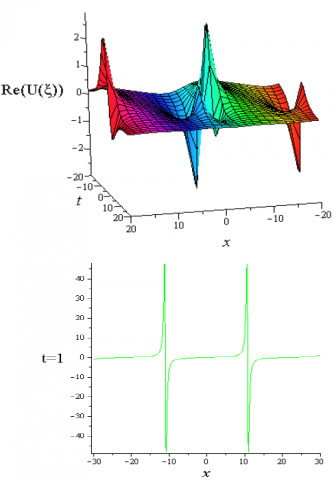Figure 1. Periodic breather wave solution of the real part of the solution $U_{3,1}$ for the parametric standards $h=-1, R=\beta=\alpha=1, \xi_{0}=-0.01$

The solutions $U_{2,1}, U_{4,1}, U_{4,2}, U_{5,1}, U_{6,1}, U_{5,2}, U_{2,1}$ represent same type solution for different values of this parameter  shown as Figure 1.

## 2.png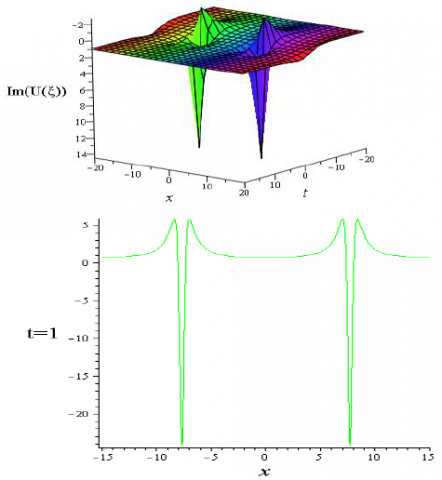Figure 2. Periodic wave solution of the imaginary part of the solution$U_{3,1}$ for the parametric standards $h=-1, R=\beta=\alpha=1, \xi_{0}=-0.01$

The solutions $U_{2,1}, U_{4,1}, U_{4,2}, U_{5,1}, U_{6,1}, U_{5,2}, U_{2,1}$ represent same type solution for different values of these parameter shown as Figure 2.

## 3.png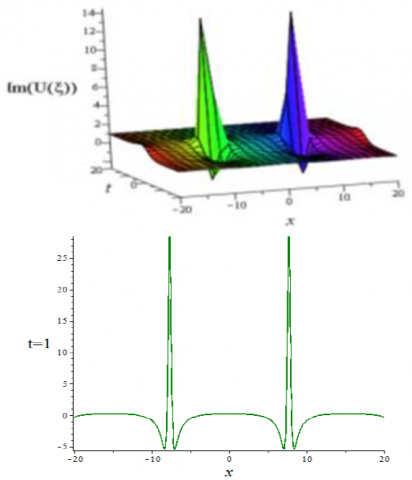Figure 3. Periodic breather wave solution of the imaginary part of the solution $U_{3,1}$ for the parametric standards $h=-1, R=\beta=1, \alpha=-1, \xi_{0}=-0.01$

The solutions $U_{1,1} U_{1,2} U_{2,1}, U_{4,1}$,

$U_{4,2}, U_{5,1}, U_{6,1}, U_{5,2}, U_{2,1}$  represent same type solution for different values of this parameter shown as Figure 3.

## 4.jpg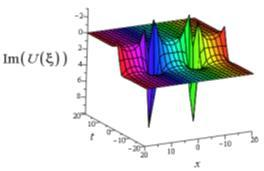Figure 4. kinky Periodic wave solution of the imaginary part of the solution $U_{3,1}$ for the parametric standardsh $=-1.5, R=1.5, \beta=1, \alpha=3, \xi_{0}=-0.01$

## 5.png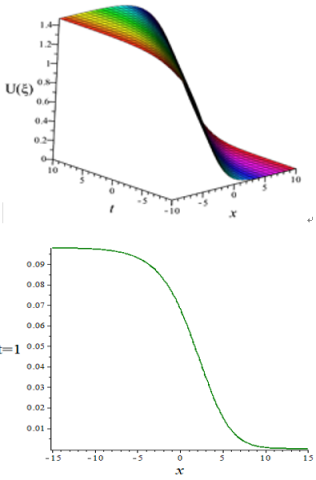Figure 5. Kink shape solution of the solution $U_{3,1}$ for the values $h=-0.2, R=\beta=\alpha=1, \xi_{0}=-0.1$

For different values of free parameters, the solutions $U_{3,2}, U_{1,1}, U_{1,2}, U_{3,3}, U_{3,4}$  show the same type solution as Figure 5.

## 6.pngFigure 6. Anti-kink shape solution of $U_{4,1}$ for the valuesh $=-0.2, R=\beta=\alpha=1, \xi_{0}=-0.1$

The solutions $U_{4,2}, U_{2,1}, U_{2,2}, U_{2,3}, U_{5,1}, U_{5,2}, U_{6,2}, U_{6,1}$  show the same type solution as Figure 6.

5. Conclusion

In this paper, the principle exertion is to discover, test and break down the new voyaging wave arrangements and physical properties of the nonlinear Oskolkov condition by applying dependable scientific procedures. The simple equation scheme performance a substantial trick to find traveling wave solutions in-terms of exponential, trigonometric and hyperbolic function from which we can build specially Kinky periodic wave, breather wave solution, solitary and periodic wave solutions. This technique offers arrangements with free parameters that may be essential to clarify some unpredictable nonlinear physical marvels.

References

 Yasar, E., Yıldırım, Y., Adem, A.R. (2018). Perturbed optical solitons with spatio-temporal dispersion in (2 + 1)-dimensions by extended Kudryashov method. Optik, 158: 1–14. https://doi.org/10.1016/j.ijleo.2017.11.205

 Roshid, H.O., Roshid, M.M., Rahman, N., Pervin, M.R. (2017). New solitary wave in shallow water, plasma and ion acoustic plasma via the GZK-BBM equation and the RLW equation. Propulsion and Power Research, 6(1): 49–57. https://doi.org/10.1016/j.jppr.2017.02.002

 Roshid, H.O., Hoque, M.F., Akbar, M.A. (2014). New extended (G’/G)-expansion method to solve nonlinear evolution equation: The (3+1) dimensional potential-YTSF equation. Springer Plus, 3: 122. https://doi.org/10.1186/2193-1801-3-122

 Feng, L.L., Zhang, T.T. (2018). Breather wave, rogue wave and solitary wave solutions of a coupled nonlinear Schrodinger equation. Appl. Math. Lett, 78: 133-140. https://doi.org/10.1016/j.aml.2017.11.011

 Xu, S.W., He, J.S. (2012). The rogue wave and breather solution of the Gerdjikov-Ivanov equation. Journal of Mathematical Physics, 53. https://doi.org/10.1063/1.4726510

 Biswas, A., Mirzazadeh, M., Eslami, M., Zhou, Q., Bhrawy, A., Belic, M. (2016). Optical solitons in nano-fibers with spatio-temporal dispersion by trial solution method. Optik, 127(18): 7250–7257. https://doi.org/10.1016/j.ijleo.2016.05.052

 Yildirim, A., Pinar, Z. (2010). Application of the Exp-function method for solving nonlinear reaction-diffusion equations arising in mathematical biology. Computers & Mathematics with Applications, 60(7): 1873-1880. https://doi.org/10.1016/j.camwa.2010.07.020

 Zhao, Y.M. (2014). New exact solutions for a higher-order wave equation of KdV type using the multiple simplest equation method. Journal of Applied Mathematics, 1-13. http://dx.doi.org/10.1155/2014/848069

 Alquran, M. (2012). Bright and dark soliton solutions to the Ostrovsky-Benjamin-Bona-Mahony (OS-BBM) equation. J. Math. Comput. Sci., 2: 15-22.

 Nofal, T.A. (2015). Simple equation method for nonlinear partial differential equations and its applications. Journal of the Egyptian Mathematical Society, 24(2): 204-209. https://doi.org/10.1016/j.joems.2015.05.006

 Bilige, S., Chaolu, T., Wang, X.M. (2013). Application of the extended simplest equation method to the coupled Schrödinger-Boussinesq equation. Applied Mathematics and Computation, 224: 517–523.  https://doi.org/10.1016/j.amc.2013.08.083

 Kudryashov, N.A. (2005). Simplest equation method to look for exact solutions of nonlinear differential equations. Chaos, Solitons & Fractals, 24(5): 1217–1231. https://doi.org/10.1016/j.chaos.2004.09.109

 Taghizadeha, N., Mirzazadeha, M., Rahimianb, M., Akbaria, M. (2013). Application of the simplest equation method to some time-fractional partial differential equations. Ain Shams Engineering Journal, 4(4): 897-902. https://doi.org/10.1016/j.asej.2013.01.006

 Amfilokhiev, V.B., Voitkunskii, Y.I., Mazaeva, N.P., Khodorkovskii, Y.S. (1975). Flows of polymer solutions in the case of convective accelerations Tr. Leningr. Korablestroit. Inst, 96: 3-9.

 Roshid, M.M., Roshid, H.O. (2018). Exact and explicit traveling wave solutions to two nonlinear evolution equations which describe incompressible viscoelastic Kelvin-Voigt fluid. Heliyon, 4. http://dx.doi.org/10.1016/j.heliyon.2018.e00756

 Gozukızıl, O.M., Akcagıl, S. (2013). The tanh-coth method for some nonlinear pseudoparabolic equations with exact solutions. Advances in Difference Equations, 143. https://doi.org/10.1186/1687-1847-2013-143

 Turgut, A.K., Aydemir, T., Saha, A., Kara, A.H. (2018). Propagation of nonlinear shock waves for the generalised Oskolkov equation and its dynamic motions in the presence of an external periodic perturbation, Pramana. J. Phys., 78-90. https://doi.org/10.1007/s12043-018-1564-7

 Sviridyuk, G.A., Shipilov, A.S. (2010). On the stability of solutions of the Oskolkov equations on a graph. Differential Equations, 46(5): 742–747. https://doi.org/10.1134/S0012266110050137

 Akcagil, S., Aydemir, T., Gozukizil, O.M. (2016). Exact travelling wave solutions of nonlinear pseudo parabolic equations by using the Expansion Method. NTMSCI, 4(4): 51-66. http://dx.doi.org/10.20852/ntmsci.2016422120

 Sviridyuk, G.A., Yakupov, M.M. (2003). The phase space of Cauchy-Dirichlet problem for a nonclassical equation. Differ. Uravn. (Minsk), 39(11): 1556-1561.